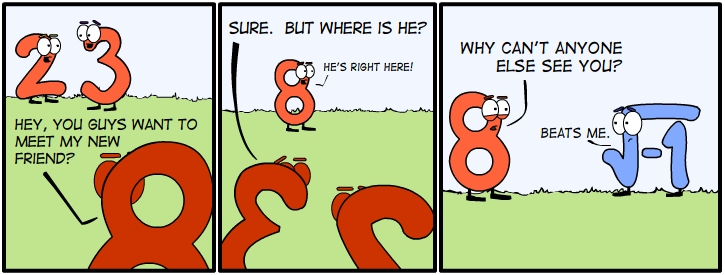M A T H 2 1 B
Mathematics Math21b Spring 2008
Linear Algebra and Differential Equations
Exhibit: other numbers
Office: SciCtr 434

## Complex numbers

A rotation dilation matrix
``` A =   | a   b |
| -b  a |
```
can be associated with a complex number z = a + i b. The matrix
``` J  = | 0   1 |
| -1  0 |
```
plays the role of the imaginary number i, because it satisfies i2 = -1. If we take two complex numbers z = a+ib, w=u+iv, then their product is z w = (au-bv) + i (av+bu). The product of the matrices
``` A B  =   | a   b |  | u   v | = | au-bv     av+bu |
| -b  a |  |-v   u |   | -(av+bu)  au-bv |
```
indeed is again a rotation dilation matrix which is associated to the complex number z w. This explanation takes a bit the mystery of the question, what is the imaginary number I because matrices allow complx numbers to be realized as a concrete object. The connection also illustrates again what the multiplication of complex numbers does: multiplying with z is a rotation and a scaling by |z|.Source: nfccomic

## Dual numbers

The set of 2x2 matrices of the form
```  | a   b |
| 0   a |
```
forms a set called dual numbers. One can add and multiply dual numbers and again gets a dual number. Similarly as in the case of complex numbers, one can write any dual number as z = a + e b. But now, instead of i2 = -1, we have the rule e2 = 0. Problem: verify that the analogue for the fundamental identity
```exp(i t) = cos(t) + i sin(t)
```
for complex numbers is
```exp(e t) = 1+ e t.
```
The use of dual numbers is motivated by infinitesimal calculus. If we think of e as an infinitesimal number, then e2 = 0. Dual numbers are also motivated from physics: if e denotes a Fermionic direction, then e2=0 is a model for the Pauli exclusion principle.

## Split complex numbers

The set of 2x2 matrices of the form
```  | a   b |
| b   a |
```
forms a set called split complex numbers. One can add and multiply split complex numbers and again get a split complex number. Similarly as in the case of complex numbers, one can write any split complex number as z = a + j b. But now, instead of i2 = -1, one has to use the rule j2 = 1. Problem: verify that the analogue for the fundamental identities for complex and dual numbers
```exp(i t) = cos(t) + i sin(t)
exp(e t) = 1+ e t.
```
is the identity
```exp(j t) = cosh(t) + j sinh(t)  .
```
The use of split complex numbers is motivated by special relativity. The correponding trnasformation is a Lorentz boost, a special Lorentz transformation.